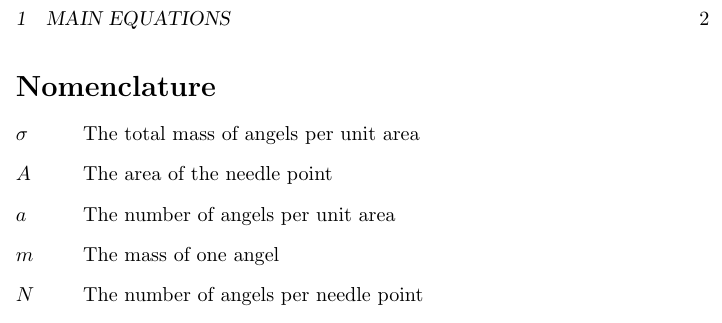\markboth changes manual running heads #2

Closed
opened this issue Jan 23, 2019 · 1 comment

Projects
None yet

komascript commented Jan 23, 2019

 For classes with chapters nomencl defines the nomenclature heading as: \chapter*{\nomname} \markboth{\nomname}{\nomname}% \if@intoc\addcontentsline{toc}{chapter}{\nomname}\fi%  The \markboth here destoys the running head, if someone use manual running heads, e.g., with page style myheadings instead of automatic running head, e.g., with page style headings: \documentclass{report} \usepackage{mwe} \usepackage[nocfg]{nomencl} \makenomenclature \pagestyle{myheadings} \markright{Example paper by KOMA} \begin{document} \chapter{Testchapter} This is a \index{test chapter} test chapter. \Blindtext \nomenclature[ex]{ex}{example} \printnomenclature \chapter{One more chapter} This could also be an index, a bibliography or something else. \Blindtext \end{document}  Without \printnomenclature the running head in the last chapter (page 5) is still "Example paper by KOMA", but with \printnomenclature it changed to "Nomenclature". So you should at least use \@mkboth instead if \markboth. BTW: It would be easier and even add extra features to nomencl if you would use package tocbasic. Here with some minor changes (nomencl.hak in KOMA-Script) would add several new features to nomencl. This would also solve another issue.

Author

komascript commented Jan 28, 2019

 The change is better, but you've to also change the article + \section* case: \documentclass{article} \usepackage[nocfg]{nomencl} \makenomenclature \pagestyle{headings} \begin{document} \section{Main equations} \begin{equation} a=\frac{N}{A} \end{equation}% \nomenclature{$a$}{The number of angels per unit area}% \nomenclature{$N$}{The number of angels per needle point}% \nomenclature{$A$}{The area of the needle point}% The equation $\sigma = m a$% \nomenclature{$\sigma$}{The total mass of angels per unit area}% \nomenclature{$m$}{The mass of one angel} follows easily. \newpage \printnomenclature \end{document}  would result inbut should result in a running head "NOMENCLATURE". BTW: Using \@mkboth{\nomname}{\@nomname} is correct for KOMA-Script classes which do not use \MakeUppercase for running heads by default, but not for the standard classes, that use upper case running heads by default unless you are using fancyhdr or scrlayer-scrpage to change this. tocbasic provides a \MakeMarkcase to handle all such cases. Try: \documentclass{article} \usepackage[nocfg,automake]{nomencl} \usepackage{tocbasic} \makenomenclature \pagestyle{headings} \begin{document} \section{Main equations} \begin{equation} a=\frac{N}{A} \end{equation}% \nomenclature{$a$}{The number of angels per unit area}% \nomenclature{$N$}{The number of angels per needle point}% \nomenclature{$A$}{The area of the needle point}% The equation $\sigma = m a$% \nomenclature{$\sigma$}{The total mass of angels per unit area}% \nomenclature{$m$}{The mass of one angel} follows easily. \newpage \printnomenclature \end{document}  with you changed or my patched version of nomencl and you will see: using tocbasic makes things easier.